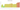GH₵

Ghanaian Cedi

how has the value of the currency changed in the last year?USD 1 = GHS 6.1342

How much is10 US dollars worth inGhanaian cedis?
At the current exchange rate, 10 US dollars is worth 61.34 Ghanaian cedis
How much is50 US dollars worth inGhanaian cedis?
At the current exchange rate, 50 US dollars is worth 306.71 Ghanaian cedis
How much is100 US dollars worth inGhanaian cedis?
At the current exchange rate, 100 US dollars is worth 613.42 Ghanaian cedis
How much is500 US dollars worth inGhanaian cedis?
At the current exchange rate, 500 US dollars is worth 3,067.12 Ghanaian cedis
How much is2,000 US dollars worth inGhanaian cedis?
At the current exchange rate, 2,000 US dollars is worth 12,268.47 Ghanaian cedis
How much is10 US dollars worth inGhanaian cedis?
At the current exchange rate, 10 US dollars is worth 61.34 Ghanaian cedis
How much is50 US dollars worth inGhanaian cedis?
At the current exchange rate, 50 US dollars is worth 306.71 Ghanaian cedis
How much is100 US dollars worth inGhanaian cedis?
At the current exchange rate, 100 US dollars is worth 613.42 Ghanaian cedis
How much is500 US dollars worth inGhanaian cedis?
At the current exchange rate, 500 US dollars is worth 3,067.12 Ghanaian cedis
How much is2,000 US dollars worth inGhanaian cedis?
At the current exchange rate, 2,000 US dollars is worth 12,268.47 Ghanaian cedis